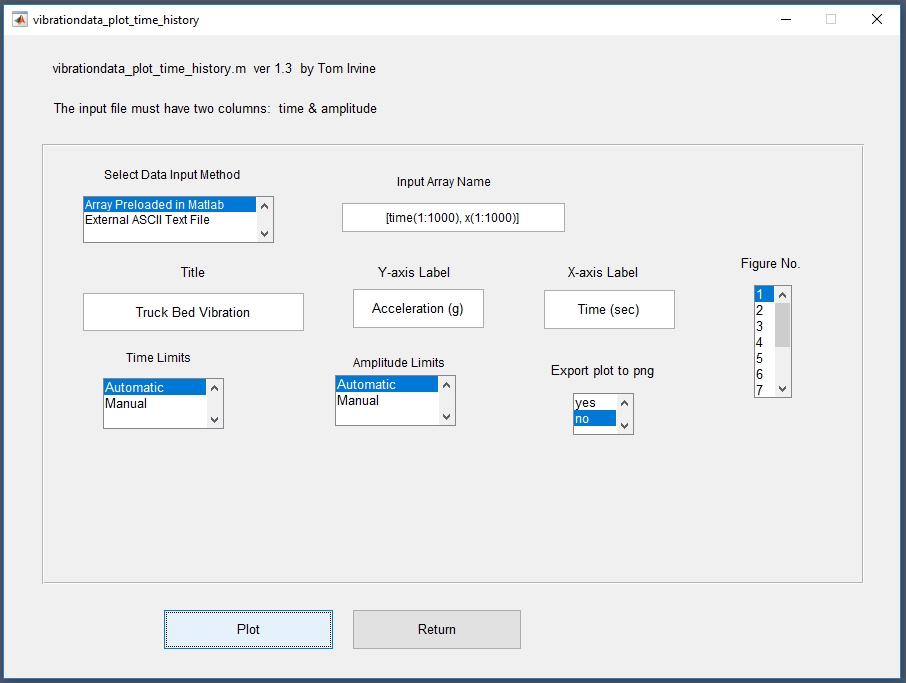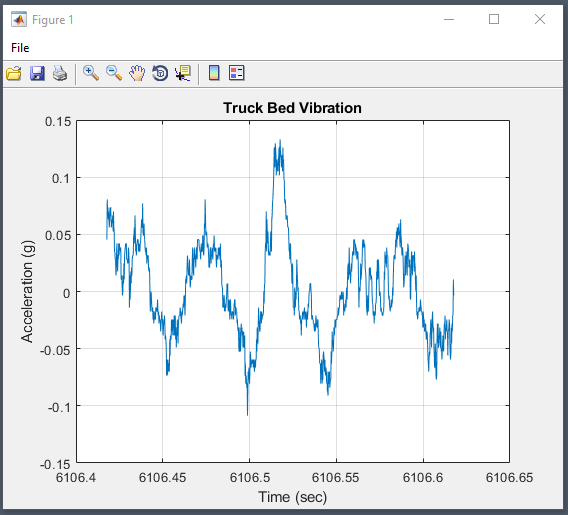# Import Data into VibrationData Toolbox

The VibrationData Toolbox is the MATLAB-compiled Signal Analysis & Structural Dynamics Package by Tom Irvine.  This article will show how to import data into the application for advanced analysis.  Visit the main site to download the toolbox to do your own analysis!

# Importing Data into the Vibration Data ToolboxThe VibrationData toolbox conveniently includes some example data files to help follow along in his tutorials that can be accessed from the bottom right of the main window.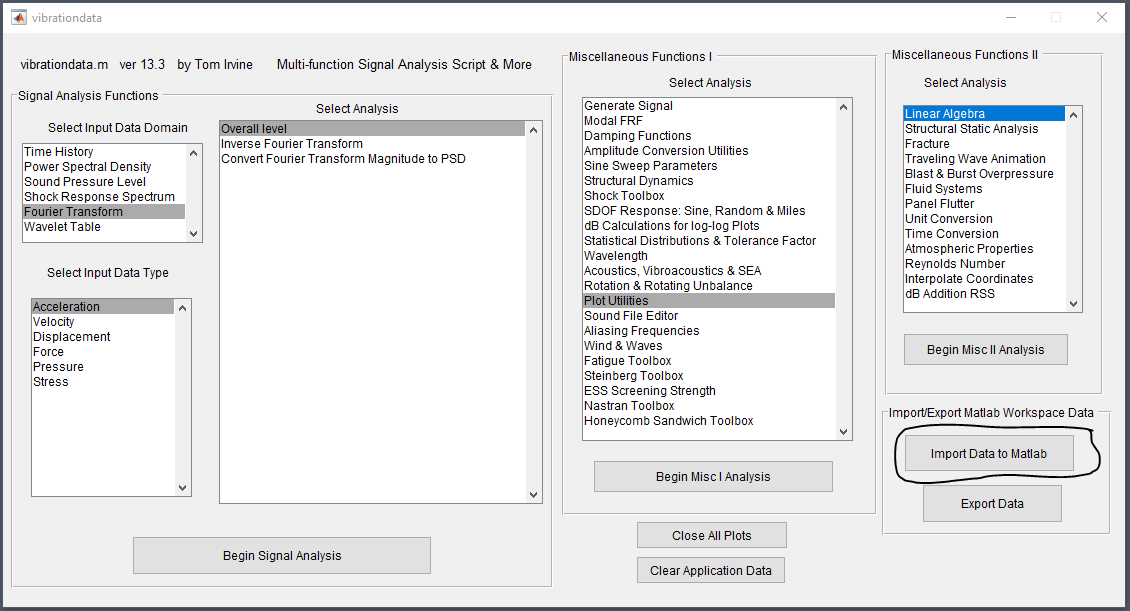The library of data sets are organized by type on the right of the next window.  This also allows for loading in external data although this requires the data to be in the format the software expects which is one matrix.  The following section and import functionality lets you load in data and define the columns as discrete variables.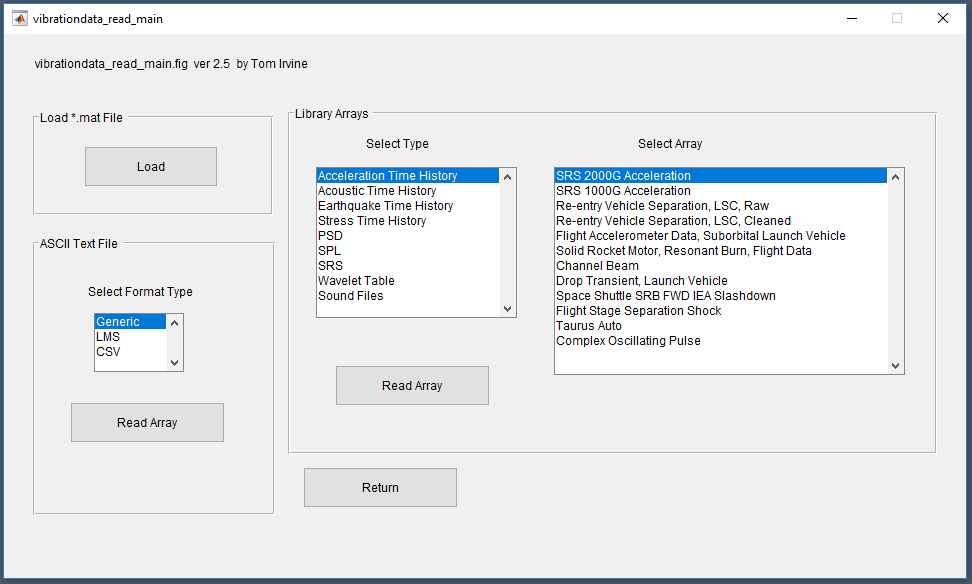To load data into the application click the "Load" button in the Workspace window.  There are several example files located where the application is installed under examples as shown.  In this example we'll load in data from vibrations taken by an enDAQ sensor on a truck bed during transit, see here for more information on the data.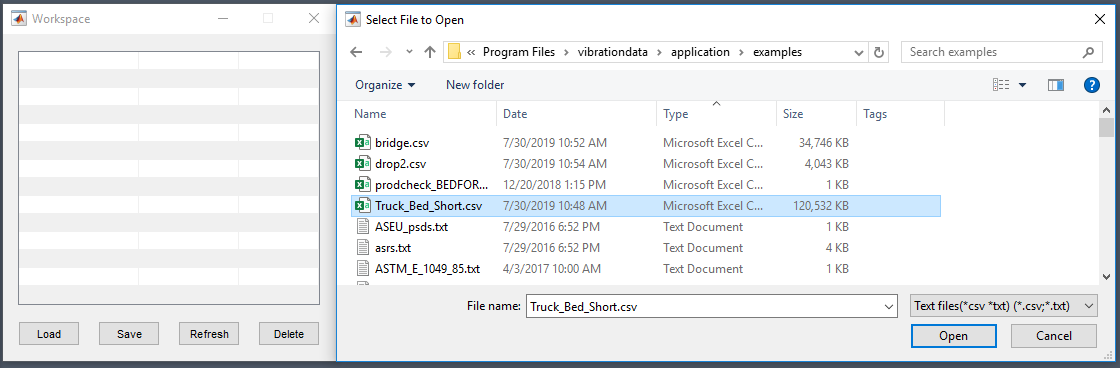You will be presented with a Load Table window as shown which will load the first 31 lines of the file you are opening.  This window will allow you to change the variable names, in this example, we've changed them from var1, var2, var3 to time, x, y.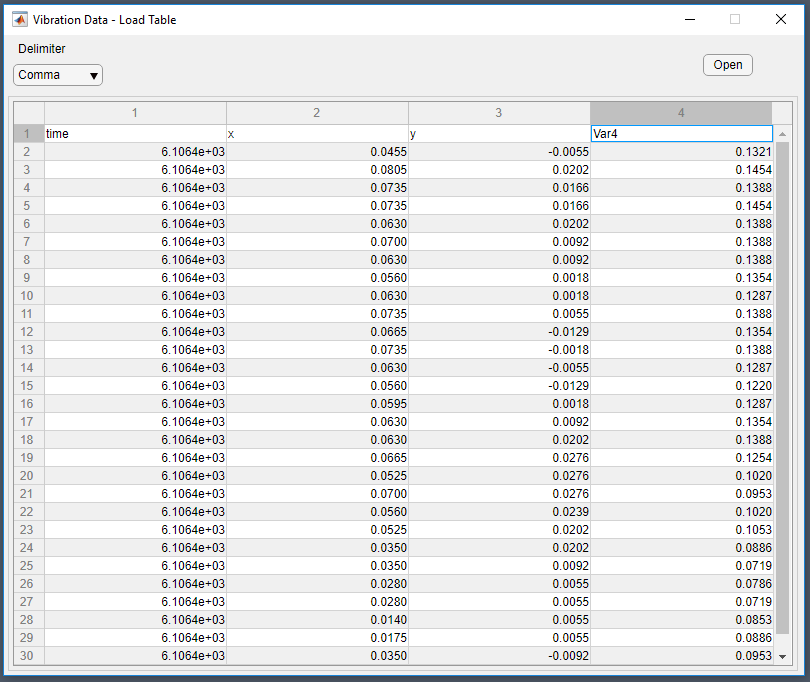You may need to hit "Refresh" which will then show you the variables in the workspace.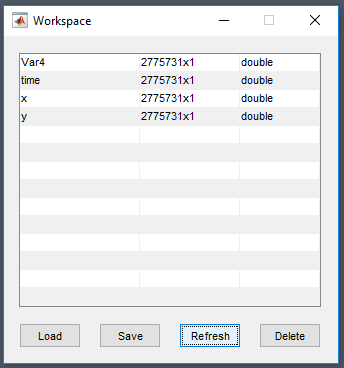# Combine Variables into Arrays

The software expects matrixes with the first column typically representing time or frequency.  This can be created from the discrete loaded variables with simple MATLAB syntax and operations:

matrix = [var1, var2]

More information on how to combine variables into matrixes is available in MATLAB's help docs.

# Simple Plotting Example

This example will show how to generate a simple plot with the loaded data.  First navigate to the Miscellaneous Functions I and select Plot Utilities.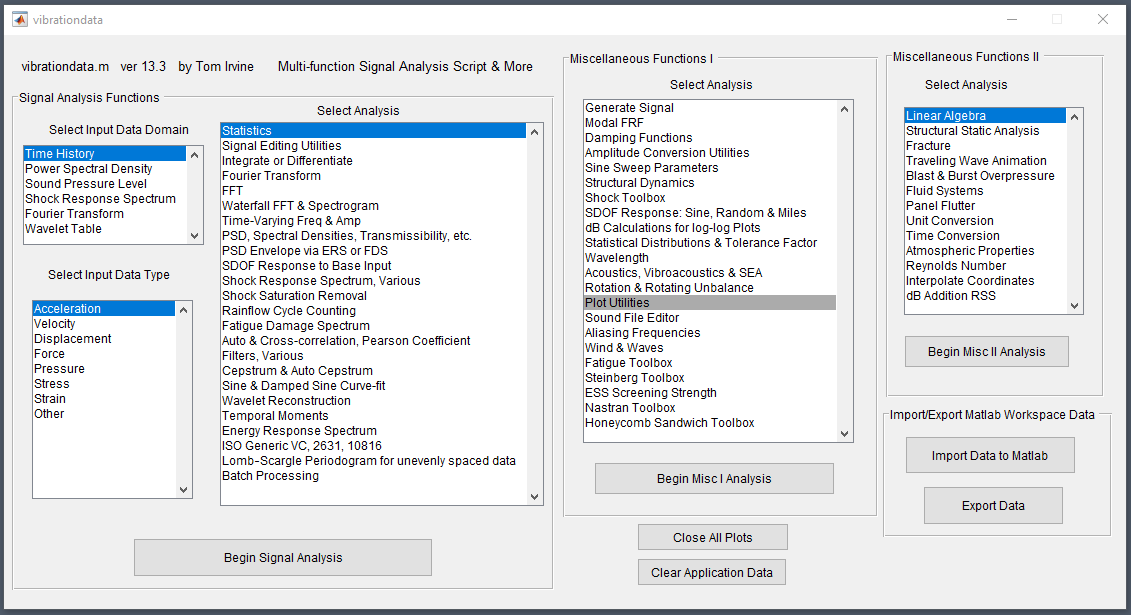You will be prompted with a follow-on window to specify the type of plot, we'll do a simple a time history.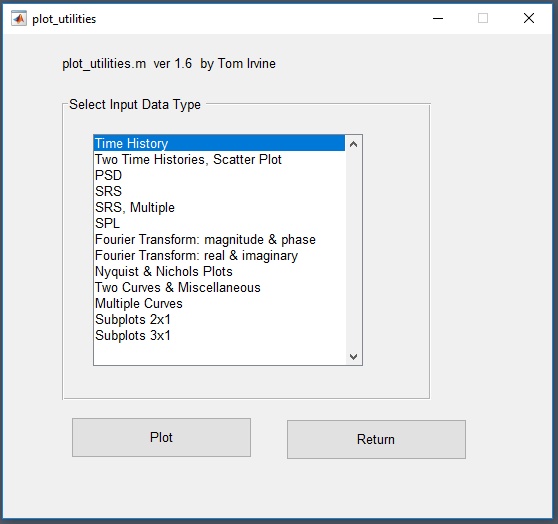Here we need to combine the variables where it asks for the Input Array Name that combines the time and x variables into a simple matrix.

[time, x]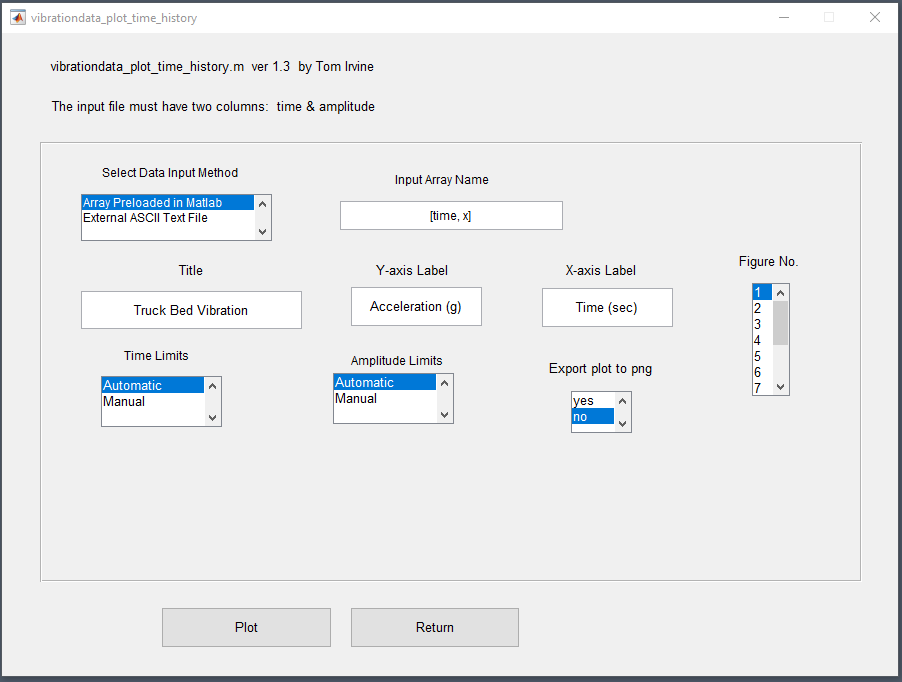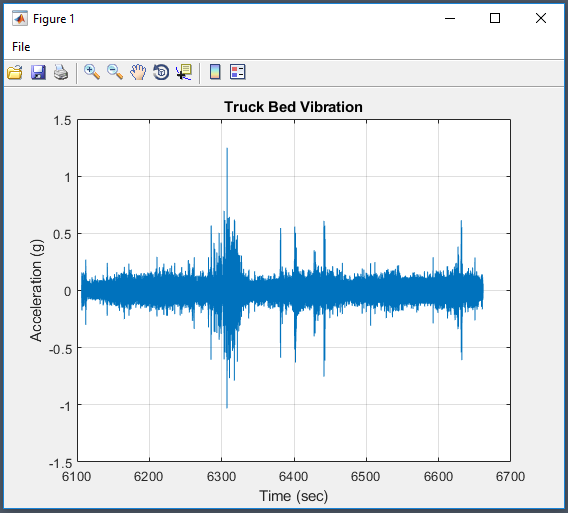We can follow this same syntax to plot a subsection of the data by specifying the range of each variable (ensuring they have the same length) as shown.  This example plots the first 1,000 data points.

[time(1:1000), x(1:1000)]